Courses

# Very Short Answer Type Questions- Coordinate Geometry Class 10 Notes | EduRev

## Class 10 : Very Short Answer Type Questions- Coordinate Geometry Class 10 Notes | EduRev

The document Very Short Answer Type Questions- Coordinate Geometry Class 10 Notes | EduRev is a part of the Class 10 Course Class 10 Mathematics by VP Classes.
All you need of Class 10 at this link: Class 10

Q1. Find a point on the y-axis equidistant from (− 5, 2) and (9, − 2).

Sol. Let the required point on the y-axis be P (0, y)

∴ PA = PB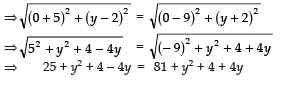⇒ y2 − y2 − 4y − 4y = 81 + 4 − 4 − 25
⇒ − 8y = 85 − 29
⇒ − 8y = 56

⇒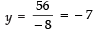∴ The required point is (0, −7).

Q2. Find a point on x-axis at a distance of 4 units from the point A (2, 1).

Sol. Let the required point on x-axis be P (x, 0).

∴ PA = 4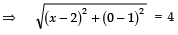⇒ x2 − 4x + 4 + 1 = 42 = 16
⇒ x2 − 4x + 1 + 4 − 16 = 0
⇒ x2 − 4x − 11 = 0

⇒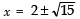Q3. Find the distance of the point (3, − 4) from the origin.

Sol. The coordinates of origin (0, 0).
∴ Distance of (3, − 4) from the origin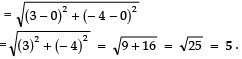Q4. For what value of x is the distance between the points A (− 3, 2) and B (x, 10) 10 units?

Sol. The distance between A (− 3, 2) and B (x, 10)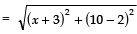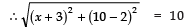⇒ (x + 3)2 + (8)2 = 102
⇒ (x + 3)2 = 102 − 82
⇒ (x + 3)2 = (10 − 8) (10 + 8) = 36
⇒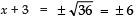For +ve sign, x =6 − 3 = 3
For −ve sign, x = − 6 − 3 = − 9

Q5. Find a point on the x-axis which is equidistant from the points A (5, 2) and B (1, − 2).
Sol.
The given points are: A (5, 2) and B (1, − 2) Let the required point on the x-axis be C (x, 0).
Since, C is equidistant from A and B.
∴ AC = BC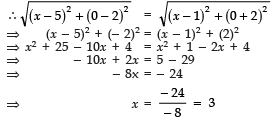∴ The required point is (0, 3).
Q6. Establish the relation between x and y when P (x, y) is equidistant from the points A (− 1, 2) and B (2, − 1).

Sol. ∵ P is equidistant from A and B
∴ PA = PB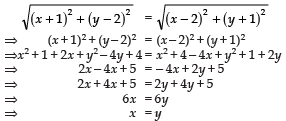which is the required relation.

Q7. Find a relation between x and y such that the point P (x, y) is equidistant from the points A (−5, 3) and B (7, 2)

Sol. Since, P (x, y) is equidistant from A (−5, 3) and B (7, 2)
∴ AP = BP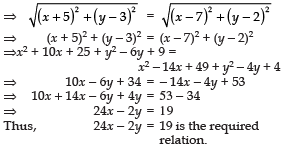Q8. Show that the points (7, − 2), (2, 3) and (− 1, 6) are collinear.

Sol. Here, the vertices of a triangle are (7, − 2), (2, 3) and (− 1, 6)
∴ Area of the triangle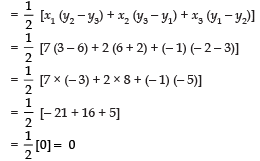Since area of triangle =  0
∴ The vertices of the triangle are collinear.
Thus, the given points are collinear.

Q9. Find the distance between the points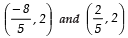Sol. Distance between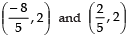is given by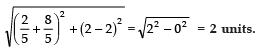Q10. If the mid point of the line joining the points P (6, b − 2) and Q (− 2, 4) is (2, − 3), find the value of b.

Sol. Here, P (6, b − 2) and Q (− 2, 4) are the given points.
∴ Mid point of PQ is given by: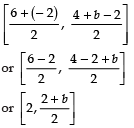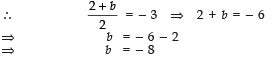Q11. In the given figure, ABC is a triangle. D and E are the mid points of the sides BC and AC respectively. Find the length of DE. Prove that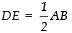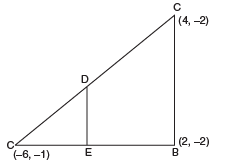Sol. Coordinates of the mid point of BC are: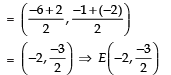Coordinates of the mid point of AC are: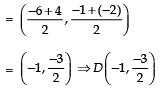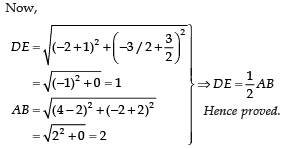Offer running on EduRev: Apply code STAYHOME200 to get INR 200 off on our premium plan EduRev Infinity!

132 docs

,

,

,

,

,

,

,

,

,

,

,

,

,

,

,

,

,

,

,

,

,

;